# Java -- 方法

1. 下列代码的运行结果为： C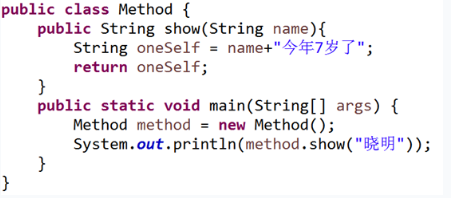A. 没有任何输出
B. 编译错误
C. 晓明今年7岁了
D. 晓明
2. 下列代码运行的结果是（） B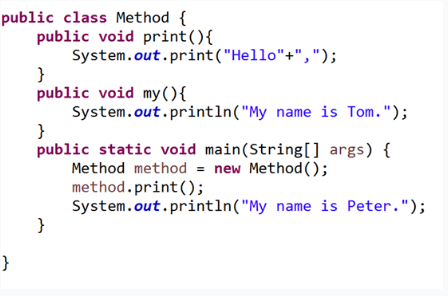A. Hello,My name is Tom.
B. Hello,My name is Peter.
C. Hello,My name is Peter.My name is Tom.
D. My name is Peter.
3. 下列语句的执行结果为（） C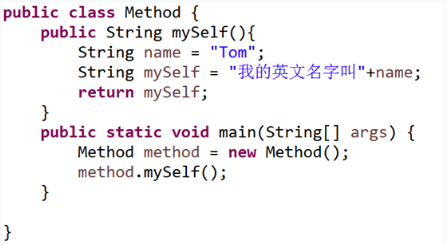A. 运行异常
B. 运行错误
C. 我的英文名字叫Tom
D. 什么都不输出
4. 下面这段程序的执行结果为： C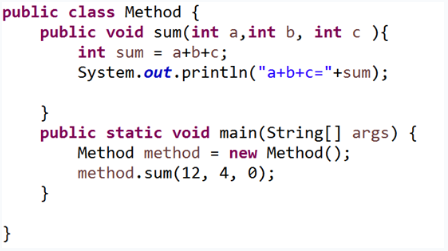A. 12
B. a+b+c=12
C. a+b+c=16
D. 16
5. 已知如下代码，则位置（1）处正确的方法调用代码为？ A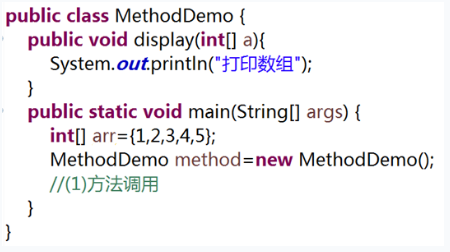A. method.display(arr);
B. method.display(arr);
C. method.display(a);
D. method.display(a);
6. 已知方法的定义形式如下：public void plus(int a,int b){}

A. public int plus(int a){}
B. public int plus1(int m,int n){}
C. public void plus(float a,float b){}
D. public int plus(int a,int b){}
7. 下列代码，前者与后者是重载关系的是： A
A. public void show(){}和public void show(int num){}
B. public void show(){}和public void print(int num){}
C. public void show(){}和public int show(){}
D. public void show(int a){}和 public void show1(int b){}
8. 以下代码的输出结果是什么？ D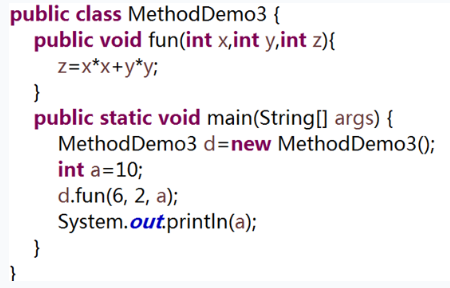A. 40
B. 8
C. 4
D. 10
9. 以下代码的输出结果是什么？ D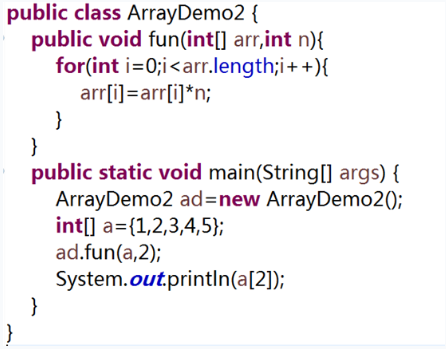A. 2
B. 3
C. 4
D. 6
10. 关于可变参数列表的描述正确的是 BC
A. 当可变参数列表作为方法参数时，不能将数组传递给可变参数列表
B. 一个方法中，只能有一个可变参数列表作为参数
C. 重载的方法中，可变参数列表作为参数的重载方法不会优先被调用
D. 数组作为方法参数时，可以将多个值同时传递给数组

1、定义一个方法，根据商品总价输出折后总价
2、在主方法中定义对象
3、使用对象调用定义的方法

public class DisCountDemo {
//根据商品总价输出折后总价
public int discount(int price) {
if(price<100) {
price=price;
}else if(price>=100&&price<200) {
price*=.95;
}else if(price>200) {
price*=.85;
}
return price;
}
//定义对象
DisCountDemo money=new DisCountDemo();
//定义商品总价存放到变量中
int price=300;
//调用方法，输出折后商品总价
int last=money.discount(price);
System.out.println(last);
}
}


1、定义一个方法，求数组的平均值
2、在主方法中定义对象，并初始化一个float类型的数组，调用方法求数组元素的平均值，并将平均值打印输出

public class AverageDemo {
//求数组元素的平均值
ublic float Average(float[] arr) {
int sum=0,a=0;
for(int i=0;i<arr.length;i++) {
sum+=arr[i];
a=sum/arr.length;
}
return a;
}
public static void main(String[] args) {
//定义对象
AverageDemo averageDemo=new AverageDemo();
//创建float类型的数组并初始化
float[] arr= new float[]{(float)1.2,(float)1.3,(float)1.4,(float)1.5};
//调用方法求平均值并打印输出
float b=averageDemo.Average(arr);
System.out.println(b);
}
}


1、 定义两个求面积的重载方法，圆周率可以使用Math.path这个常量代替
2、在主方法中调用方法并输出

public class AreaDemo {
//求圆形面积
public double circle(double bj) {
double area=3.14*bj*bj;
return area;
}

//求长方形面积
public float oblong(double a,double b) {
double area=a*b;
return (float)area;
}

public static void main(String[] args) {
//定义对象
//定义一个double类型的变量存放半径，并初始化
//定义两个变量存放长和宽，并初始化
double a=3,b=4,r=3;
//调用方法，求圆的面积并打印输出
//调用方法，求长方形面积并打印输出
double c=(double)(area.circle(r)),d=area.oblong(a, b);
System.out.println(c);
System.out.println(d);
}
}